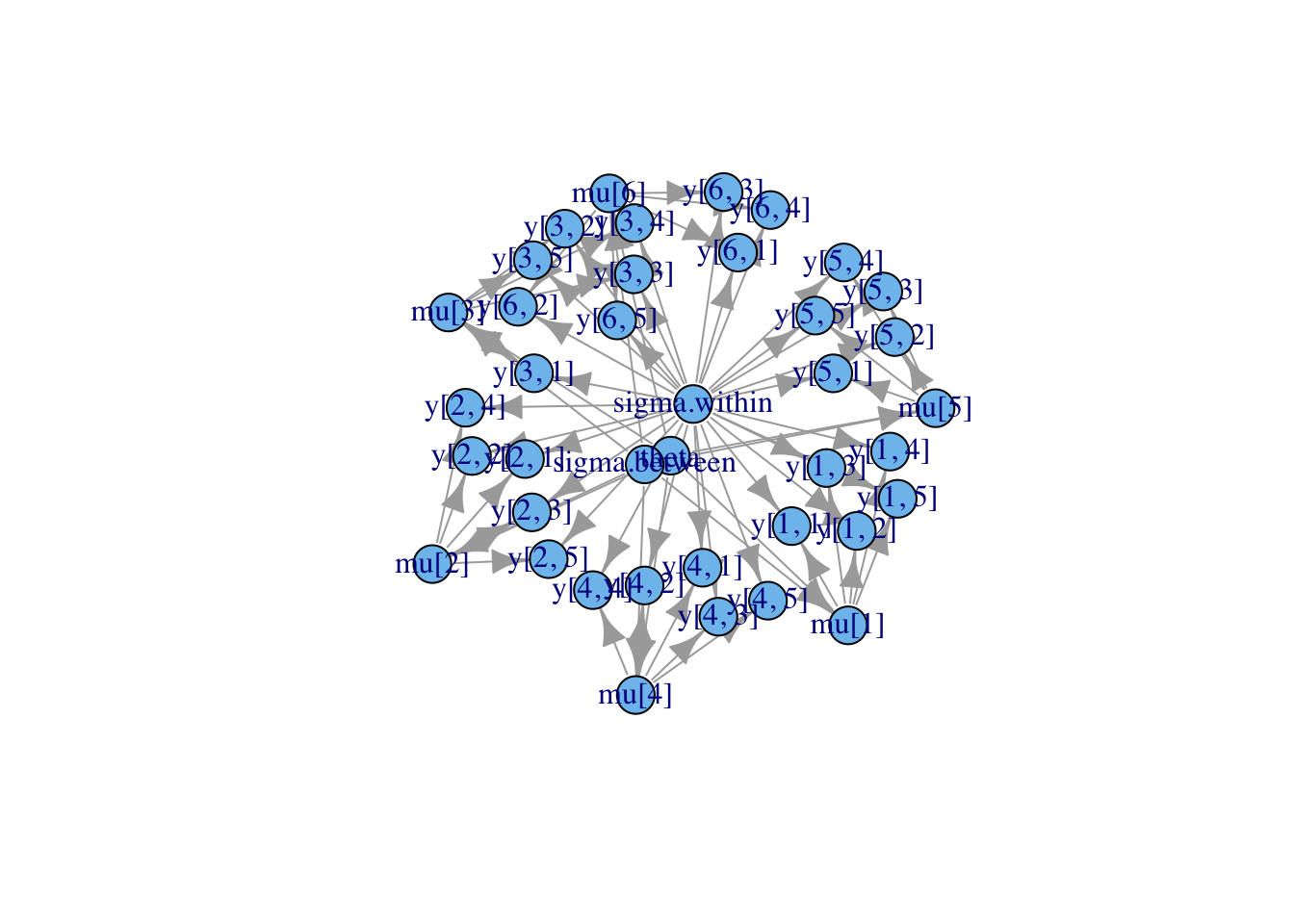# Building a model from BUGS code in R using NIMBLE

The BUGS language for declaring statistical models was popularized by WinBUGS, OpenBUGS and JAGS. Those generate and run an MCMC for the model, but they don’t allow a programmer to use the model in any other way. NIMBLE provides a new implementation of the BUGS language and creates model objects you can program with.

NIMBLE accommodates most of BUGS, but it doesn’t yet handle stochastic indices (indices that are random variables). It also extends the BUGS language in a bunch of ways that we won’t go into here. See the User Manual for more details.

## Dyes example

Let’s pick a simple model from the classic WinBUGS examples: the dyes example. This is a simple normal hierarchical model. A description can be found here. A copy of the original is on our GitHub repository here. A modified version we will use is set up like this:

library(nimble)

dyesCode <- nimbleCode({
for (i in 1:BATCHES) {
for (j in 1:SAMPLES) {
y[i,j] ~ dnorm(mu[i], sd = sigma.within);
}
mu[i] ~ dnorm(theta, sd = sigma.between);
}

theta ~ dnorm(0.0, 1.0E-10);
sigma.within ~ dunif(0, 100)
sigma.between ~ dunif(0, 100)
})

Compared to the original, this has been modified by using standard deviation parameters instead of precision parameters in two places – for the sole purpose of illustrating NIMBLE’s ability to handle different parameterizations – and removing the posterior predictive nodes.

By the way, any of the standard WinBUGS examples can be loaded automatically in NIMBLE like this:

classicDyesModel <- readBUGSmodel('dyes', dir = getBUGSexampleDir('dyes'))

## Create the model

We can create a model from dyesCode like this:

dyesModel <- nimbleModel(dyesCode, constants = list(BATCHES = 6, SAMPLES = 5))
## defining model...
## building model...
## checking model...   (use nimbleModel(..., check = FALSE) to skip model check)
## NAs were detected in model variables: theta, logProb_theta, sigma.within, logProb_sigma.within, sigma.between, logProb_sigma.between, mu, logProb_mu, y, logProb_y.
## model building finished

And we can set data values in it like this:

data <- matrix(c(1545, 1540, 1595, 1445, 1595, 1520, 1440, 1555, 1550,
1440, 1630, 1455, 1440, 1490, 1605, 1595, 1515, 1450, 1520, 1560,
1510, 1465, 1635, 1480, 1580, 1495, 1560, 1545, 1625, 1445), nrow = 6)
dyesModel$setData(list(y = data)) dyesModel$y
##      [,1] [,2] [,3] [,4] [,5]
## [1,] 1545 1440 1440 1520 1580
## [2,] 1540 1555 1490 1560 1495
## [3,] 1595 1550 1605 1510 1560
## [4,] 1445 1440 1595 1465 1545
## [5,] 1595 1630 1515 1635 1625
## [6,] 1520 1455 1450 1480 1445

## Use the model

Now we can use the model like an R object.

### Set or get values

dyesModel$theta <- 1500 dyesModel$mu <- rnorm(6, 1500, 50)
dyesModel$sigma.within <- 20 dyesModel$sigma.between <- 20
dyesModel$mu ##  1538 1435 1534 1579 1572 1430 dyesModel$y[1,]
##  1545 1440 1440 1520 1580

### Calculate log probability densities for part or all of the model

## arbitrary example
calculate(dyesModel, c('theta', 'mu[1:6]', 'y[,2]'))
##  -148

### Simulate part or all of the model

## arbitrary example
simulate(dyesModel, c('mu[1:3]'))
dyesModel$mu ##  1511 1458 1526 1579 1572 1430 ### Query the model’s relationships ## arbitrary example dyesModel$getDependencies(c('theta', 'mu'))
##   "theta"   "mu"   "mu"   "mu"   "mu"   "mu"   "mu"
##   "y[3, 1]" "y[3, 2]" "y[3, 3]" "y[3, 4]" "y[3, 5]"

### Plot the model graph (thanks to igraph’s plot feature)

library(igraph)
plot(dyesModel$getGraph())## Compile the model Finally we can compile the model and use the compiled version the same way we used the uncompiled version above: compiled_dyesModel <- compileNimble(dyesModel) compiled_dyesModel$theta <- 1450
calculate(compiled_dyesModel) ## all nodes by default
##  -383.4

Naturally, the compiled version is much faster.